### Transmission Line Model: Short and Medium lines

In last few articles we discussed some basic design aspects of electric transmission lines. Sometimes the discussion about a certain part was very brief considering that the same can be elaborated more somewhere else. Effort is made to flash the important points or parts of the subject.

In the previous 3/4 articles we developed some concepts on  AC transmission line parameters and discussed how the line parameters can be calculated under different configuration of phase conductors. The behavior of transmission lines and its voltage and current under normal and abnormal conditions depends on these parameters. For carrying out system studies the proper modelling of transmission lines  is very important. Here in this article we discuss the transmission line modeling . Transmission lines are also called as power lines. Transmission line theory is important for both power and electronics or communication  engineering. But here our discussion is mainly from the power engineering point of view.

The transmission lines are mainly classified as short, medium or long lines. The classification is based upon the accuracy of the model and simplicity. While the short line model is quite simple, the long line model is somewhat complex and the medium line is in-between the two. At power frequency of 60 or 50 Hz, the lines of length below 80 km are treated as short lines and the length of line exceeding 80 km but less than 250 km can be modeled as medium lines and as the length of lines exceed 250 km it is long line.

For short and medium length lines the parameters are lumped. This simplifies the model and gives quite accurate result. In case of the long transmission lines the line parameters are considered as distributed along the length of the line. The aim of the transmission line model is that it should be simple and the analysis of the model should bear desired accuracy. Of course you can apply the long line theory for power lines of length below 80 km. Doing so one can achieve somewhat more accurate result (which may not be desired) while making the model or calculation more complex.

Here in this article we will discuss short and medium length lines only and long line is the topic of the next article.

Before proceeding further, as we already discussed in our previous articles there are four line parameters, these are line resistance  R , inductance L, leakage conductance G and line to neutral capacitance C per meter length of line. Here instead of using the symbol Cn as the capacitance between line and neutral we will adopt C.

Here we will use these.
z = Series impedance per meter length = R + j ωL    Ω / meter    (ω = 2 π f   where f is the frequency)

y = Shunt admittance per meter length = G + j ωC   S / meter

Ω symbol is for Ohm, the unit of impedance
S symbol is for siemens, the unit of admittance. mho or inverted Ohm symbol is also used for

As already discussed in our previous article G arises due to leakage currents and is ignored in transmission line modelling.

so,  y =  j ωC   S / meter

If,  Z is the total lumped line impedance and Y is the total admittance between the line and neutral, then we get Z and Y by multiplying per meter values with the total length of the line. Then
Z  =  z .l       Ω
Y  =  y .l       S

where l is the total length of the line in meter.

Short Lines

As already said the lines of below 80 km length can be modeled as short lines. The transmission line is a three phase system and here assumed as balanced due to transposition and balanced load at receiving end. The analysis adopted here can be applied to unbalanced non-transposed systems for obtaining result with reasonable accuracy. For the balanced three phase line we are only required to analyze one phase. The return path is imaginary neutral.
In Fig-A is shown a short transmission line. The resistance and inductance are  lumped together which is the transmission line serie impedance Z.  Further for short line here the capacitance of the line is ignored so the admittance is ignored.

As shown the sending end and receiving end voltages are Vs and Vr respectively and the sending end and receiving end currents are Is and Ir respectively.

From the diagram it is clear that both sending and receiving end current are the same
Is = Ir
According to Kirchhoff's voltage law,
Vs = Vr  + Z Ir

Z = R + j XL
R is the total line resistance and XL is the total line inductance, both are lumped.

Those who are familiar with two port network analysis, the above can be represented in terms of ABCD parameters. In this form the supply side voltage and current is represented in terms of receving side voltage and current as below

Vs = A Vr  + B Ir
I = C Vr  + D Ir
In the above formulas A and D are dimension less. Dimension of B is Ohm and that of C is Siemens.

The parameters satisfy the condition   AD-BC =1

In transmission line problem this representation is sometimes found suitable. Usually the receiving side voltage and current requirement are given and it is required to find the supply side voltage and current.

For the above short line equations we get
A = 1,  B=Z, C=0,  D=1

clearly it satisfies the condition AD-BC=1

Voltage Regulation
The performance of a transmission line is determined by a term called voltage regulation. Voltage regulation is defined as the percentage change in voltage at the receiving end from No load to Full load  with sending end voltage held constant.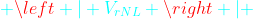or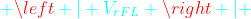means the values or magnitudes of  voltages without sign . So the subtraction is the simple subtraction and not the phasor subtraction. The voltage regulation is desired to be small or zero.  Integrated Grids has set standards  for voltage variation say  ±5% or ±7% depending on voltage levels.

The relationship  Vs = Vr  + Z Ir   between the sending end and receiving end voltages can also be illustrated by a phasor diagram shown in Fig-B. In Fig-B(i) we have considered an inductive load. So the receiving end current  Ir   lags the receiving end voltage Vr by a phase angle φ(determined by load). In Fig-B(ii) is considered a capacitive load. Here current  Ir  leads the voltage Vby an angle φ.

In both the diagrams Vr  and  Z Ir   are added as per the rule of phasor addition. Z is the line impedance as discussed above.

Z I = (R+j XL ) I = R Ir   +j XL I

The main difference between the above two is that in case of lagging load the receiving end voltage is less than the sending end voltage. But in case of leading load the receiving end voltage can even become more than the sending end voltage depending on the lead phase angle φ . Which is due to the fact that the load is capacitive. In our case Fig-B (ii), for the chosen phase angle the receiving end voltage is more than the sending end voltage. Actually the industrial loads are mostly inductive. The inductive loads consume reactive power. Capacitors which generate reactive power are connected at the load end or at substations to supply reactive power. At sub-transmission and distribution levels where voltage drop is a problem, capacitors can improve the voltage profile and power factor at the receiving end. The capacitive load helps in improving the receiving end voltage regulation and power factor. For only voltage regulation purpose usually tap changer of transformer is utilized. By tap changing the transformer turns ratio is changed, so adjusting the secondary voltage.

Another common definition which sometimes may be useful for evaluation of transmission line performance is the transmission efficiency of the line. The transmission efficiency is defined as the ratio of power delivered at receiving end to the power supplied at sending end.

If η is the transmission efficiency then,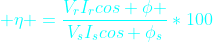cos φ  is the power factor at the receiving end, or power factor of load but  cos φ is the power factor at the sending end (due to both load and transmission line).
Using the above formula it is easy to find the transmission efficiency of short and medium lines. For short line the angle φs is clearly visible from the phasor diagram (angle between  Vs and Is (or Ir)). But in case of medium line little more effort is required.

Medium Lines

The medium length lines are categorized as transmission lines of more than 80 km and less than 250 km length. The medium length lines can be modeled two ways. These are nominal π and nomial T (Fig-C), so named due to the arrangement of elements. Due to the length of the  line admittance Y is no more neglected. Of course in both the π and nomial T representations lumped elements are used.  In the nominal π representation the total admittance due to line capacitance is equally divided into two halves and each half connected at both sending and receiving ends. In the nominal T representation the admittance is connected at the center of line and line impedance is divided into two equal parts and each part is connected on both sides in series (Fig-C).

It can be shown that for the π representation the sending end voltage and current can be written in terms of receiving end voltage and current as shown below.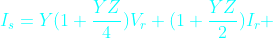From above two expressions ABCD parameters can be easily identified. Also you should verify the condition AD-BC=1.

For T representation also applying KCL and KVL we can obtain the similar expression for Vs and Is.

At no load putting I= 0   we obtain Vr NL  = V/ A, .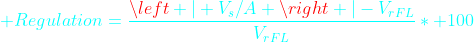Analysis of short and medium lines helps us gain important concepts of transmission lines. It is useful for long line analysis.

tammy said...

hmmm interesting sketch

Pos Systems
Pos Systemamna said...

nice:)amna said...

good info i like itAnonymous said...

thanx for this info

Unknown said...

very less information...

Audience Response System said...

This one article alone shows off your excellent writing skills.

Audience Response System said...

Is there any smart people left in this universe?saurabh said...

mast and thanks

CPS Clicker said...

Do you have any additional articles on the same topic?

Whichschool Magazine said...

SEO Specialist in the Philippines said...

Hi. I wanted to drop you a quick word to express my thanks. Ive been following your weblog for a month or so and have picked up a ton of excellent info and loved the tactic youve structured your site. I am trying to run my very own weblog nonetheless I believe its too normal and I need to deal with a lot of smaller topics. Being all things to all folks is not all that its cracked up to be.

Bow Point Nursery said...

wo wo wooooah! this blog is very helpful and effective, i expand my knowledge in this field more. Great work!

Jaiper Feliciano said...

I really enjoyed reading this post. I congratulate you for the terrific job you’ve made. Great stuff, just simply amazing!

My Deaf America said...

Wonderful work! This is the type of information that should be shared around the internet. Shame on the search engines for not positioning this post higher! Come on over and visit my site . Thanks =)

Cochrane Ranchehouse said...

Wow,great content and your blog template is so beautiful. Is this template free or not. If so, would you please share this template? if not, how much does it cost? Thanks a lot!

Unknown said...

good ...nice infoAnonymous said...

Why haven't we taken Vs as refrence in both nominal π and nominal T circuit?

Unknown said...

Why we give supply to both side means sending end as well as receiving end

Bella said...

At receiving end there is not a suppy it is a load which is represented as supply.

Unknown said...

thanks you for this information and i give for Every one this site for booking
http://fliphtml5.com/lnym/jdfy/basic/151-200

arolincy said...

Nice blog was really feeling good to read it. Thanks for this information.
Spoken English Classes in Chennai
English Speaking Course in Chennai
french classes
pearson vue test center in chennai
IoT Training in Chennai
Xamarin Training in Chennai
Node JS Training in Chennai
Spoken English Classes in OMR
Spoken English Classes in Porur